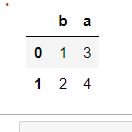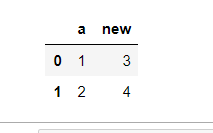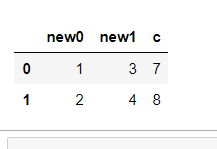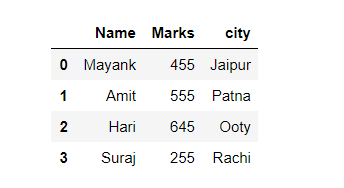Rename column by index in Pandas

• Last Updated : 26 Dec, 2020

Prerequisites: Pandas

A column of a data frame can be changed using the position it is in known as its index. Just by the use of index a column can be renamed. This article discusses all such possible methods.

Approach:

• Import required python library.
• Create data
• Provide index of the column to be renamed as argument to rename() function.

Pandas rename() method is used to rename any index, column or row.

Syntax: rename(mapper=None, index=None, columns=None, axis=None, copy=True, inplace=False, level=None)

Parameters:

• mapper, index and columns: Dictionary value, key refers to the old name and value refers to new name. Only one of these parameters can be used at once.
• axis: int or string value, 0/’row’ for Rows and 1/’columns’ for Columns
• copy: Copies underlying data if True.
• inplace: Makes changes in original Data Frame if True.
• level: Used to specify level in case data frame is having multiple level index.

Return Type: Data frame with new names

Given below are various implementation to achieve our required functionality:

Example 1: Switching both column names with each other using column index.

Python3

 import pandas as pd  # Sample DataFramedf = pd.DataFrame({'a': [1, 2], 'b': [3, 4]})  # Changing columns name with index numberdf.columns.values = "b"df.columns.values = "a"  # Displaydisplay(df)

Output:Example 2: Using another method to rename the column with index.

Python3

 import pandas as pd  # Sample DataFramedf = pd.DataFrame({'a': [1, 2], 'b': [3, 4]})  # Changing columns name with index numbersu = df.rename(columns={df.columns: 'new'})  # Displaydisplay(su)

Output:Example 3: Renaming two/more columns in a single command using an index number.

Python3

 import pandas as pd  # Sample DataFramedf = pd.DataFrame({'a': [1, 2], 'b': [3, 4], 'c': [7, 8]})  # Changing columns name with index numbermapping = {df.columns: 'new0', df.columns: 'new1'}su = df.rename(columns=mapping)  # Displaydisplay(su)

Output:Example 4: Renaming column name with an index number of the CSV file.

File in use: Data1.csv